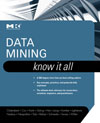# Calculating mode in data mining projects

## Learn tips for data mining, including how to work with data rollup for sums and averages, and learn about calculating mode, the key to event-driven architecture (EDA), in this tutorial from the book Data Mining: Know it All.Data Mining: Know it All

In this chapter from Data Mining: Know it All, learn learn how to calculate the average value or the number of records that represent certain events during the data rollup process.

### 2.5 Rollup with Sums, Averages, and Counts

In addition to finding the sum of a value variable during the rollup, it may also be more meaningful sometimes to calculate average value or the number of records that represent certain events -- for example, number of deposits, number of withdrawals, or number of mailings a customer received responding to an offer.

An introduction to data mining

Fielded applications of data mining and machine learning

The difference between machine learning and statistics in data mining

Information and examples on data mining and ethics

Data acquisition and integration techniques

What is a data rollup?

Calculating mode in data mining projects

Using data merging and concatenation techniques to integrate data

In our rollup macro, these requirements would alter only the middle part of our code, where we calculated the cumulative value of the Value variable. The following code segment would modify the macro to calculate the average value and the number of transactions for each account type instead of the total:

Step 2
data Temp1;
retain TOT 0;
retain NT 0;
set & TDS;
by & IDVar & TimeVar & TypeVar;
if fi rst. & TypeVar then TOT = 0;
TOT = TOT + & Value;
if & Value ne . then NT = NT + 1;
if last. & TypeVar then
do;
AVG = TOT/ NT;
output;
NT = 0;
end;
drop & Value;
run;

Furthermore, the code inside the %do loop should also reflect our interest in transposing the values of the average variable, _AVG. Therefore, the code will be as follows:

Step 4
%do i = 1 %to & N;
0proc transpose
data = Temp1
out = R & i
prefi x = %substr( & & Cat & i, 1, & Nchars) ;
by & IDVar & TypeVar;
ID & TimeVar;
var AVG;
where & TypeVar = " & & Cat & i " ;
run;
%end;

The complete code for the modified code to roll up the average value is included in the macro ABRollup() .

### 2.6 Calculation of the Mode

Another useful summary statistic is the mode, which is used in both the rollup stage and the event-driven architecture (EDA). The mode is the most common category of transaction. The mode for nominal variables is equivalent to the use of the average or the sum for the continuous case. For example, when customers use different payment methods, it may be beneficial to identify the payment method most frequently used by each customer.

The computation of the mode on the mining view entity level from a transaction dataset is a demanding task because we need to search for the frequencies of the different categories for each unique value of the entity variable. The macro shown in Table 2.5 is based on a classic SQL query for finding the mode on the entity level from a transaction table. The variable being searched is XVar, and the entity level is identified through the unique value of the variable IDVar:

%macro VarMode(TransDS, XVar, IDVar, OutDS);
/ * A classic implementation of the mode of transactional
data using SQL * /
proc sql noprint;
create table & OutDS as

Table 2.5 Parameters of VarMode ( ) Macro

 Header Parameter VarMode (TransDS, XVar, IDVar, OutDS) Description TransDS Input transaction dataset XVar Variable for which the mode is to be calculated IDVar ID variable OutDS The output dataset with the mode for unique IDs

SELECT & IDVar , MIN( & XVar ) AS mode
FROM (
SELECT & IDVar, & XVar
FROM & TransDS p1
GROUP BY & IDVar, & XVar
HAVING COUNT( * ) =
(SELECT MAX(CNT )
FROM (SELECT COUNT( * ) AS CNT
FROM & TransDS p2
WHERE p2. & IDVar = p1. & IDVar
GROUP BY p2. & XVar
) AS p3
)
) AS p
GROUP BY p. & IDVar;
quit;
%mend;

The query works by calculating a list holding the frequency of the XVar categories, identified as CNT, then using the maximum of these counts as the mode. The query then creates a new table containing IDVar and XVar where the XVar category frequency is equal to the maximum count, that is, the mode.

#### More on data mining:

The preceding compound SELECT statement is computationally demanding because of the use of several layers of GROUP BY and HAVING clauses. Indexing should always be considered when dealing with large datasets. Sometimes it is even necessary to partition the transaction dataset into smaller datasets before applying such a query to overcome memory limitations.

Close